### GATE 2014 ECE Video Solutions on EDC (Electronic Devices and Circuits)

Set – 1 (15th February 2014 (Forenoon))

1. When the optical power incident on a photo diode is 10 µW and the responsivity is 0.8 A/W, the photo current generated (in µA) is ____________.
Answer: 8 µA
Solution : https://www.youtube.com/watch?v=FdWts4kUgOI

2. In the figure, assume that the forward voltage drops to the PN diode D1 and Schottky diode D2 are 0.7 volts and 0.3 volts respectively. If ON denotes conducting state of the diode and OFF denotes the non conducting state of the diode, then in the circuit,a. Both are ON
b. D1 is ON and D2 is OFF
c. Both are OFF
d. D1 is OFF and D2 is ON

Answer: D
Solution : https://www.youtube.com/watch?v=qteoesfhVco

3. If the fixed positive charges are present in the gate oxide of an N channel enhancement type MOSFET, it will lead to
a. a decrease in the threshold voltage
b. channel length modulation
c. an increase in substrate leakage current
d. an increase in accumulation capacitance

Answer: A
Solution : https://www.youtube.com/watch?v=em0VvbA7po4

4. A good current buffer has
a. Low input impedance and low output impedance
b. Low input impedance and high output impedance
c. high input impedance and low output impedance
d. high input impedance and high output impedance

Solution : https://www.youtube.com/watch?v=vdYmByP9zYY

5. A BJT is biased in forward active mode. Assume VBE = 0.7 volts, KT/q = 25 mV and reverse saturation current IS = 10-13 Amp. The Transconductance of the BJT (in mA/volt) is…..

Solution : https://www.youtube.com/watch?v=nAh0aTdVp84

6. The doping concentration on the P side and N side of a silicon diode are 1x1016 cm-3 and 1x1017 cm-3 respectively. A forward bias of 0.3 volts is applied to the diode. At T = 300oK, the intrinsic carrier concentration of silicon, ni = 1.5 x 1010 cm-3 and KT/q = 26 mV. The electron concentration at the edge of the depletion region on the P side is
a. 2.3 x 109 cm-3
b. 1 x 1016 cm-3
c. 1 x 1017 cm-3
d. 2.25 x 106 cm-3

Solution : https://www.youtube.com/watch?v=KJ1NDAVBw0U

7. A depletion type N channel MOSFET is biased in its linear region for use as a voltage controlled resistor. Assume threshold voltage VTH = -0.5 volts, VGS = 2.0 volts, VDS = 5 volts, W/L = 100, Cox = 10-8 F/cm2 and µn = 800 cm2/volt-sec. The value of the voltage controlled resistor (in Ω) is …..

Solution : https://www.youtube.com/watch?v=xzUDuBbZUXM

SET – 2 (15th February 2014 (Afternoon))

1. A silicon bar is doped with donor impurities ND = 2.25 x 1015 atoms/cm3. Given the intrinsic carrier concentration of silicon at T = 300oK is ni = 1.5 x 1010 cm-3. Assuming complete impurity ionization, the equilibrium electron and hole concentrations are
a. no = 1.5 x 1016 cm-3, p0 = 1.5 x 105 cm-3
b. no = 1.5 x 1010 cm-3, p0 = 1.5 x 1015 cm-3
c. no = 1.5 x 1015 cm-3, p0 = 1.5 x 1010 cm-3
d. no = 1.5 x 1015 cm-3, p0 = 1.5 x 105 cm-3

Solution : https://www.youtube.com/watch?v=zvQFdUBhINg

2. an increase in the base recombination of a BJT will increase
a. the common emitter DC current gain, β
b. the breakdown voltage BVCEO
c. the unity gain cutoff frequency, fτ
d. the Transconductance gm

Solution : https://www.youtube.com/watch?v=mY6YLyFzlyc

3. In CMOS technology, shallow P – well or N – well regions can be formed using
a. low pressure chemical vapor deposition
b. low energy sputtering
c. low temperature dry oxidation
d. low energy ion – implantation

Solution : https://www.youtube.com/watch?v=NA-HnsA124g

4. Assume electron charge q = 1.6 x 10-19C, KT/q = 25 mV and electron mobility µn = 1000 cm2/volt-sec. If the concentration gradient of electrons injected into a P type silicon sample is 1 x 1021 per cm-3, the magnitude of electron diffusion current density (in A/cm2) is ………….

Solution : https://www.youtube.com/watch?v=IBb_zfgQ1AY

5. Consider an abrupt PN junction (at T = 300oK) shown in the figure. The depletion region width Xn on the N side of the junction is 0.2 µm and the permittivity of silicon (Ԑsi) is 1.044 x 10-12 F/cm. At the junction, the approximate value of the peak electric field (in kV/cm) is………..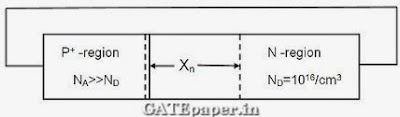Solution : https://www.youtube.com/watch?v=6SGWS0QE-dQ

6. When a silicon diode having a doping concentration of NA = 9 x 1016 cm-3 on P side and ND = 1 x 1016 cm-3 on N side is reversed biased, the total depletion width is found to be 3 µm. Given that the permittivity of silicon is 1.04 x 10-12 F/cm, the depletion width on the P side and the maximum electric field in the depletion region, respectively, are
a. 2.7 µm and 2.3 x 105 V/cm
b. 0.3 µm and 4.15 x 105 V/cm
c. 0.3 µm and 0.42 x 105 V/cm
d. 2.1 µm and 0.42 x 105 V/cm

Solution : https://www.youtube.com/watch?v=PRyI9roqNbA

7. The diode in the circuit shown, if Von = 0.7 volts but is ideal otherwise. If Vi = 5 sin(ωt) volts, the minimum and maximum values of Vo (in volts) are, respectively,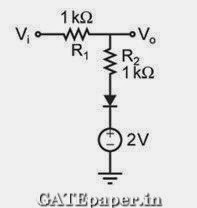a. -5 and 2.7
b. 2.7 and 5
c. -5 and 3.85
d. 1.3 and 5

Solution : https://www.youtube.com/watch?v=VNI1bsbW2i8

8. For the N channel MOS transistor shown in the figure, the threshold voltage VTH is 0.8 volts. Neglect channel length modulation effects. When the drain voltage VD = 1.6 volts, the drain current ID was found to be 0.5 mA. If VD is adjusted to be 2 volts by changing the values of R and VDD, the new value of ID (in mA) is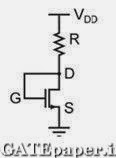a. 0.625
b. 0.75
c. 1.125
d. 1.5

Solution : https://www.youtube.com/watch?v=wsv90UUqYVY

9. For the MOSFETs shown in the figure, the threshold voltage |Vt| = 2 volts and K = 0.5µcox(W/L) = 0.1 mA/V2. The value of ID (in mA) is …………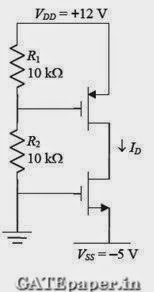Solution : https://www.youtube.com/watch?v=abv9XXV0jks

SET – 3 (16th February 2014 (Forenoon))

1. In MOSFET fabrication, the channel length is defined during the process of
a. Isolation oxide growth
b. Channel stop implantation
c. Poly-silicon gate patterning
d. Lithography step leading to the contact pads

Solution : https://www.youtube.com/watch?v=QPQ5hOdbGjE

2. A thin P type silicon sample is uniformly illuminated with light which generates excess carriers. The recombination rate is directly proportional to
a. The minority carrier mobility
b. The minority carrier recombination lifetime
c. The majority carrier concentration
d. The excess minority carrier concentration

Solution : https://www.youtube.com/watch?v=47L011RUiuM

3. At T = 300oK, the hole mobility of a semiconductor µp = 500 cm2/volt-sec and KT/q = 26 mV. The hole diffusion constant Dp in cm2/sec is ………

Solution : https://www.youtube.com/watch?v=cfegvwvHk-A

4. In the circuit shown, the PNP transistor has |VBE| = 0.7 volts and β = 50. Assume that RB = 100 KΩ. For Vo to be 5 volts, the value of RC (in KΩ) is ……..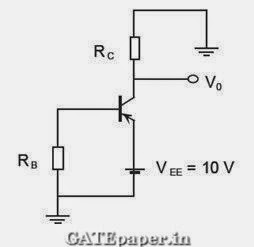Solution : https://www.youtube.com/watch?v=ohf5m4r0gzs

5. The donor and acceptor impurities in an abrupt junction silicon diode are 1 x 1016 cm-3 and 5 x 1018 cm-3, respectively. Assume that the intrinsic carrier concentration in silicon ni = 1.5 x 1010 cm-3 at 300oK, KT/q = 26 mV and the permittivity of silicon Ԑsi = 1.04 x 10-12 F/cm. The built in potential and the depletion width of the diode under thermal equilibrium conditions, respectively are
a. 0.7 volts and 1 x 10-4 cm
b. 0.86 volts and 1 x 10-4 cm
c. 0.7 volts and 3.3 x 10-5 cm
d. 0.86 volts and 3.3 x 10-5 cm

Solution : https://www.youtube.com/watch?v=W1tmcbDY0-4

6. The slope of the ID vs. VGS curve of an N channel MOSFET in linear region is 10-3-1 at VDS = 0.1 volts. For the same device, neglecting channel length modulation, the slope of the √ID vs. VGS curve (in √A/V) under saturation regime is approximately…………….

Solution : https://www.youtube.com/watch?v=rDjsCvkSUtk

7. An ideal MOS capacitor has boron doping concentration of 1015 cm-3 in the substrate. When a gate voltage is applied, a depletion region of width 0.5 µm is formed with a surface (channel) potential of 0.2 volts. Given that Ԑo = 8.854 x 10-14 F/cm and the relative permittivity’s of silicon and silicon dioxide are 12 and 4 respectively. The peak electric field (in V/µm) in the oxide region is ……………..

Solution : https://www.youtube.com/watch?v=K4aRAJxegSI

8. In the circuit shown, the silicon BJT has β = 50. Assume VBE = 0.7 volts and VCEsat = 0.2 volts. Which one of the following statements is correct?a. For RC = 1 kΩ, the BJT operates in the saturation region
b. For RC = 3 kΩ, the BJT operates in the saturation region
c. For RC = 20 kΩ, the BJT operates in the cutoff region
d. For RC = 20 kΩ, the BJT operates in the linear region

Solution : https://www.youtube.com/watch?v=zd_NFD2CN8E

9. For the MOSFET M1 shown in the figure, assume W/L = 2, VDD = 2.0 volts, µncox = 100 µA/V2 and VTH = 0.5 volts. The transistor M1 switches from saturation region to linear region when Vin (in volts) is …………..Solution : https://www.youtube.com/watch?v=AWdemWJenw4

SET - 4 (16th February 2014 (Afternoon))

1. At T = 300oK, the band gap and the intrinsic carrier concentration of GaAs are 1.42 eV and 106 cm-3 respectively. In order to generate electron hole pairs in GaAs, which one of the wavelength (λc) ranges of incident radiation, is most suitable? (Given that Plank’s constant = 6.62 x 10-34 J-sec, velocity of light is 3 x 1010 cm/s and charge of electron is 1.6 x 10-19C)
a. 0.42 µm < λc < 0.87 µm
b. 0.87 µm < λc < 1.42 µm
c. 1.42 µm < λc < 1.62 µm
d. 1.62 µm < λc < 6.62 µm

Solution : https://www.youtube.com/watch?v=NML_fLbqHxw

2. In the figure, ln(ρi) is plotted as a function of 1/T, where ρi is the intrinsic resistivity of silicon, T is the temperature, and the plot is almost linear. The slope of the line can be used to estimatea. Band gap energy of silicon
b. Sum of electron and hole mobility in silicon
c. Reciprocal of the sum of electron and hole mobility in silicon
d. Intrinsic carrier concentration of silicon

Solution : https://www.youtube.com/watch?v=nqt3J_lXwuU

3. The cutoff wavelength (in µm) of light that can be used for intrinsic excitation of a semiconductor material of band gap Eg = 1.1 eV is …………..

Solution : https://www.youtube.com/watch?v=bfF2NoqjjDU

4. If the emitter resistance in a common emitter voltage amplifier is not bypassed, it will
a. Reduce both the voltage gain and the input impedance
b. Reduce the voltage gain and increase the input impedance
c. Increase the voltage gain and reduce the input impedance
d. Increase both the voltage gain and the input impedance

Solution : https://www.youtube.com/watch?v=4YjclPDw5lM

5. Consider a silicon sample doped with ND = 1 x 1015 /cm3 donor atoms. Assume that the intrinsic carrier concentration ni = 1.5 x 1010 cm-3. If the sample is additionally doped with NA = 1 x 1018 cm-3 acceptor atoms, the approximate number of electrons /cm3 in the sample, at T = 300oK, will be…………

Solution : https://www.youtube.com/watch?v=T4s49hCIrz0

6. Consider two BJTs biased at the same collector current with area A1 = 0.2 µm x 0.2 µm and A2 = 300 µm x 300 µm. assuming that all other device parameters are identical, KT/q = 26 mV, the intrinsic carrier concentration is 1 x 1010 cm-3, and q = 1.6 x 10-19C, the difference between the base emitter voltages (in mV) of the two BJTs (i.e. VBE1 – VBE2) is …………….

Solution : https://www.youtube.com/watch?v=R-GogUWvN74

7. An N type semiconductor having uniform doping is biased as shown in the figure.Solution : https://www.youtube.com/watch?v=XhOs_vAWETw

8. A BJT in a common base configuration is used to amplify a signal received by a 50Ω antenna. Assume KT/q = 25 mV, the value of collector bias current (in mA) required to match the input impedance of the amplifier to the impedance of the antenna is ………..

Solution : https://www.youtube.com/watch?v=HBjqJb91WbE

Name

Email *

Message *